# PSAT Math : How to find the surface area of a prism

## Example Questions

### Example Question #11 : Prisms

A rectangular prism has a volume of 70 m3.  If the length, width, and height of the prism are integers measured in meters, which of the following is NOT a possible measure of the surface area of the prism measured in square meters?

178

174

118

280

214

280

Explanation:

Since the volume is the product of length, width, and height, and each of these three dimensions are integers, it is important to know the factors of the volume.  70 = (2)(5)(7).  This implies that each of these factors (and only these factors with the exception of 1) will show up in the three dimensions exactly once.  This creates precisely the following five possibilities:

2, 5, 7

SA = 2((2)(5)+(2)(7)+(5)(7)) = 118

1, 7, 10

SA = 2((1)(7)+(1)(10)+(7)(10)) = 174

1, 5, 14

SA = 2((1)(5)+(1)(14)+(5)(14)) = 178

1, 2, 35

SA = 2((1)(2)+(1)(35)+(2)(35)) = 214

1, 1, 70

SA = 2((1)(1)+(1)(70)+(1)(70)) = 282

### Example Question #1 : How To Find The Surface Area Of A Prism

The three sides of a rectangular box all have integer unit lengths. If each of the side lengths is greater than one unit, and if the volume of the box is 182 cubic units, what is the surface area of the box in square units?

264

181

262

182

236

262

Explanation:

Let's call the side lengths of the box l, w, and h. We are told that l, w, and h must all be integer lengths greater than one. We are also told that the volume of the box is 182 cubic units.

Since the volume of a rectangular box is the product of its side lengths, this means that lwh must equal 182.

(l)(w)(h) = 182.

In order to determine possible values of l, w, and h, it would help us to figure out the factors of 182. We want to express 182 as a product of three integers each greater than 1.

Let's factor 182. Because 182 is even, it is divisible by 2.

182 = 2(91).

91 is equal to the product of 7 and 13.

Thus, 182 = 2(7)(13).

This means that the lengths of the box must be 2, 7, and 13 units.

In order to find the surface area, we can use the following formula:

surface area = 2lw + 2lh + 2hw.

surface area = 2(2)(7) + 2(2)(13) + 2(7)(13)

= 28 + 52 + 182

= 262 square units.

### Example Question #1 : How To Find The Surface Area Of A Prism

A right rectangular prism has dimensions of 3 x 5 x 20. What is its surface area?

175

56

112

350

300

350

Explanation:

There are six faces to a right, rectangular prism. Based on our dimensions, we know that we must have a face that is 3 x 5, a face that is 5 x 20 and a face that is 3 x 20. To think this through, imagine that the front face is 3 x 5, the right side is 5 x 20, and the top is 3 x 20. Now, each of these sides has a matching side opposite (the left has the right, the top has the bottom, the front has the back).

Therefore, we know we have the following areas for the faces of our prism:

2 * 3 * 5 = 30

2 * 5 * 20 = 200

2 * 3 * 20 = 120

Add these to get the total surface area:

30 + 200 + 120 = 350

### Example Question #1 : How To Find The Surface Area Of A Prism

A right rectangular prism has dimensions of 12.4 x 2.3 x 33. What is its surface area?

1882.32

1027.24

470.58

941.16

513.62

1027.24

Explanation:

There are six faces to a right, rectangular prism. Based on our dimensions, we know that we must have a face that is 12.4 x 2.3, a face that is 2.3 x 33 and a face that is 33 x 12.4. To think this through, imagine that the front face is 12.4 x 2.3, the left side is 2.3 x 33, and the top is 33 x 12.4. Now, each of these sides has a matching side opposite (the left has the right, the top has the bottom, the front has the back).

Therefore, we know we have the following areas for the faces of our prism:

2 * 12.4 * 2.3 = 57.04

2 * 2.3 * 33 = 151.8

2 * 12.4 * 33 = 818.4

Add these to get the total surface area:

57.04 + 151.8 + 818.4 = 1027.24

### Example Question #5 : How To Find The Surface Area Of A Prism

The dimensions of a right rectangular prism are such that the second dimension is twice the length of the first and the third is twice the length of the second. If the volume of the prism is 216 cubic units, what is its surface area?

189 square units

126 square units

215 square units

252 square units

252 square units

Explanation:

Based on our prompt, we can say that the prism has dimensions that can be represented as:

Dim1: x

Dim2: 2 * Dim1 = 2x

Dim3: 2 * Dim2 = 2 * 2x = 4x

More directly stated, therefore, our dimensions are: x, 2x, and 4x. Therefore, the volume is x * 2x * 4x = 216, which simplifies to 8x3 = 216 or x3 = 27. Solving for x, we find x = 3. Therefore, our dimensions are:

x = 3

2x = 6

4x = 12

Or: 3 x 6 x 12

Now, to find the surface area, we must consider that this means that our prism has sides of the following dimensions: 3 x 6, 6 x 12, and 3 x 12. Since each side has a "matching" side opposite it, we know that we have the following values for the areas of the faces:

2 * 3 * 6 = 36

2 * 6 * 12 = 144

2 * 3 * 12 = 72

The total surface area therefore equals: 36 + 144 + 72 = 252 square units.

### Example Question #6 : How To Find The Surface Area Of A Prism

The area of a given object is 30,096 in2. What is the area of this object in ft2?

1881 ft2

2508 ft2

209 ft2

1254 ft2

209 ft2

Explanation:

Converting squared units is not difficult, though you have to be careful not to make a simple mistake. It is tempting to think you can merely divide the initial value (30,096) by 12, as though you were converting from inches to feet.

Begin by thinking this through as follows. In the case of a single dimension, we know that:

1 ft = 12 in   or   1 in = (1/12) ft

Now, think the case of a square with dimensions 1 ft x 1 ft. This square has the following dimensions in inches: 12 in x 12 in. The area is therefore 12 * 12 = 144 in2. This holds for all two-dimensional conversions. Therefore, the two dimensional conversion equation is:

1 ft2 = 144 in2   or   1 in2 = (1/144) ft2

Based on this, we can convert our value 30,096 in2 thus: 30,096/144 = 209 ft2.

### Example Question #1 : How To Find The Surface Area Of A Prism

The area of a given object is 24 yd2. What is the area of this object in in2?

864 in2

20,736 in2

10,368 in2

31,104 in2

31,104 in2

Explanation:

Converting squared units is not difficult, though you have to be careful not to make a simple mistake. It is tempting to think you can merely multiply the initial value (24) by 36, as though you were converting from yards to inches.

Begin by thinking this through as follows. In the case of a single dimension, we know that:

1 yd = 36 in

Now, think the case of a square with dimensions 1 yd x 1 yd. This square has the following dimensions in inches: 36 in x 36 in. The area is therefore 36 * 36 = 1296 in2. This holds for all two-dimensional conversions. Therefore, the two dimensional conversion equation is:

1 yd2 = 1296 in2

Based on this, we can convert our value 24 yd2 thus: 24 * 1296 = 31,104 in2.

### Example Question #1 : How To Find The Surface Area Of A Prism

Angie is painting a 2 foot cube for a play she is in. She needs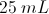of paint for every square foot she paints. How much paint does she need?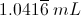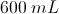It is impossible to convert between metric units and feet.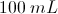Explanation:

First we must calculate the surface area of the cube. We know that there are six surfaces and each surface has the same area: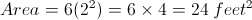Now we will determine the amount of paint needed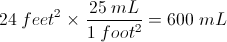### Example Question #2 : How To Find The Surface Area Of A Prism

What is the surface area of an equilateral triangluar prism with edges of 6 in and a height of 12 in?

Let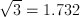and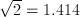.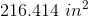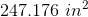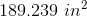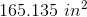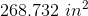Explanation:

The surface area of the prism can be broken into three rectangular sides and two equilateral triangular bases.

The area of the sides is given by: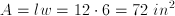, so for all three sides we get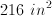.

The equilateral triangle is also an equiangular triangle by definition, so the base has congruent sides of 6 in and three angles of 60 degrees.  We use a special right traingle to figure out the height of the triangle: 30 - 60 - 90.  The height is the side opposite the 60 degree angle, so it becomes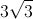or 5.196.

The area for a triangle is given by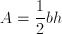and since we need two of them we get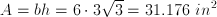.

Therefore the total surface area is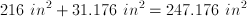.

### Example Question #101 : Solid Geometry

What is the total surface area of a box without a lid, if the dimensions of the base of the box are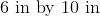, and the box is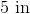tall?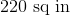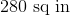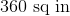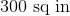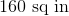The surface area of a rectangular prism is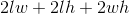. Our prism, however, is missing its top, so it will be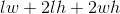. This gives us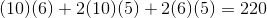.